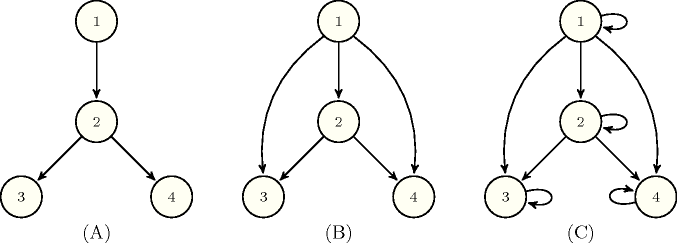## 5.134. dom_reachability

Origin
Constraint

$\mathrm{𝚍𝚘𝚖}_\mathrm{𝚛𝚎𝚊𝚌𝚑𝚊𝚋𝚒𝚕𝚒𝚝𝚢}\left(\begin{array}{c}\mathrm{𝚂𝙾𝚄𝚁𝙲𝙴},\hfill \\ \mathrm{𝙵𝙻𝙾𝚆}_\mathrm{𝙶𝚁𝙰𝙿𝙷},\hfill \\ \mathrm{𝙳𝙾𝙼𝙸𝙽𝙰𝚃𝙾𝚁}_\mathrm{𝙶𝚁𝙰𝙿𝙷},\hfill \\ \mathrm{𝚃𝚁𝙰𝙽𝚂𝙸𝚃𝙸𝚅𝙴}_\mathrm{𝙲𝙻𝙾𝚂𝚄𝚁𝙴}_\mathrm{𝙶𝚁𝙰𝙿𝙷}\hfill \end{array}\right)$

Arguments
 $\mathrm{𝚂𝙾𝚄𝚁𝙲𝙴}$ $\mathrm{𝚒𝚗𝚝}$ $\mathrm{𝙵𝙻𝙾𝚆}_\mathrm{𝙶𝚁𝙰𝙿𝙷}$ $\mathrm{𝚌𝚘𝚕𝚕𝚎𝚌𝚝𝚒𝚘𝚗}\left(\mathrm{𝚒𝚗𝚍𝚎𝚡}-\mathrm{𝚒𝚗𝚝},\mathrm{𝚜𝚞𝚌𝚌}-\mathrm{𝚜𝚟𝚊𝚛}\right)$ $\mathrm{𝙳𝙾𝙼𝙸𝙽𝙰𝚃𝙾𝚁}_\mathrm{𝙶𝚁𝙰𝙿𝙷}$ $\mathrm{𝚌𝚘𝚕𝚕𝚎𝚌𝚝𝚒𝚘𝚗}\left(\mathrm{𝚒𝚗𝚍𝚎𝚡}-\mathrm{𝚒𝚗𝚝},\mathrm{𝚜𝚞𝚌𝚌}-\mathrm{𝚜𝚒𝚗𝚝}\right)$ $\mathrm{𝚃𝚁𝙰𝙽𝚂𝙸𝚃𝙸𝚅𝙴}_\mathrm{𝙲𝙻𝙾𝚂𝚄𝚁𝙴}_\mathrm{𝙶𝚁𝙰𝙿𝙷}$ $\mathrm{𝚌𝚘𝚕𝚕𝚎𝚌𝚝𝚒𝚘𝚗}\left(\mathrm{𝚒𝚗𝚍𝚎𝚡}-\mathrm{𝚒𝚗𝚝},\mathrm{𝚜𝚞𝚌𝚌}-\mathrm{𝚜𝚟𝚊𝚛}\right)$
Restrictions
 $\mathrm{𝚂𝙾𝚄𝚁𝙲𝙴}\ge 1$ $\mathrm{𝚂𝙾𝚄𝚁𝙲𝙴}\le |\mathrm{𝙵𝙻𝙾𝚆}_\mathrm{𝙶𝚁𝙰𝙿𝙷}|$ $\mathrm{𝚛𝚎𝚚𝚞𝚒𝚛𝚎𝚍}$$\left(\mathrm{𝙵𝙻𝙾𝚆}_\mathrm{𝙶𝚁𝙰𝙿𝙷},\left[\mathrm{𝚒𝚗𝚍𝚎𝚡},\mathrm{𝚜𝚞𝚌𝚌}\right]\right)$ $\mathrm{𝙵𝙻𝙾𝚆}_\mathrm{𝙶𝚁𝙰𝙿𝙷}.\mathrm{𝚒𝚗𝚍𝚎𝚡}\ge 1$ $\mathrm{𝙵𝙻𝙾𝚆}_\mathrm{𝙶𝚁𝙰𝙿𝙷}.\mathrm{𝚒𝚗𝚍𝚎𝚡}\le |\mathrm{𝙵𝙻𝙾𝚆}_\mathrm{𝙶𝚁𝙰𝙿𝙷}|$ $\mathrm{𝙵𝙻𝙾𝚆}_\mathrm{𝙶𝚁𝙰𝙿𝙷}.\mathrm{𝚜𝚞𝚌𝚌}\ge 1$ $\mathrm{𝙵𝙻𝙾𝚆}_\mathrm{𝙶𝚁𝙰𝙿𝙷}.\mathrm{𝚜𝚞𝚌𝚌}\le |\mathrm{𝙵𝙻𝙾𝚆}_\mathrm{𝙶𝚁𝙰𝙿𝙷}|$ $\mathrm{𝚍𝚒𝚜𝚝𝚒𝚗𝚌𝚝}$$\left(\mathrm{𝙵𝙻𝙾𝚆}_\mathrm{𝙶𝚁𝙰𝙿𝙷},\mathrm{𝚒𝚗𝚍𝚎𝚡}\right)$ $\mathrm{𝚛𝚎𝚚𝚞𝚒𝚛𝚎𝚍}$$\left(\mathrm{𝙳𝙾𝙼𝙸𝙽𝙰𝚃𝙾𝚁}_\mathrm{𝙶𝚁𝙰𝙿𝙷},\left[\mathrm{𝚒𝚗𝚍𝚎𝚡},\mathrm{𝚜𝚞𝚌𝚌}\right]\right)$ $|\mathrm{𝙳𝙾𝙼𝙸𝙽𝙰𝚃𝙾𝚁}_\mathrm{𝙶𝚁𝙰𝙿𝙷}|=|\mathrm{𝙵𝙻𝙾𝚆}_\mathrm{𝙶𝚁𝙰𝙿𝙷}|$ $\mathrm{𝙳𝙾𝙼𝙸𝙽𝙰𝚃𝙾𝚁}_\mathrm{𝙶𝚁𝙰𝙿𝙷}.\mathrm{𝚒𝚗𝚍𝚎𝚡}\ge 1$ $\mathrm{𝙳𝙾𝙼𝙸𝙽𝙰𝚃𝙾𝚁}_\mathrm{𝙶𝚁𝙰𝙿𝙷}.\mathrm{𝚒𝚗𝚍𝚎𝚡}\le |\mathrm{𝙳𝙾𝙼𝙸𝙽𝙰𝚃𝙾𝚁}_\mathrm{𝙶𝚁𝙰𝙿𝙷}|$ $\mathrm{𝙳𝙾𝙼𝙸𝙽𝙰𝚃𝙾𝚁}_\mathrm{𝙶𝚁𝙰𝙿𝙷}.\mathrm{𝚜𝚞𝚌𝚌}\ge 1$ $\mathrm{𝙳𝙾𝙼𝙸𝙽𝙰𝚃𝙾𝚁}_\mathrm{𝙶𝚁𝙰𝙿𝙷}.\mathrm{𝚜𝚞𝚌𝚌}\le |\mathrm{𝙳𝙾𝙼𝙸𝙽𝙰𝚃𝙾𝚁}_\mathrm{𝙶𝚁𝙰𝙿𝙷}|$ $\mathrm{𝚍𝚒𝚜𝚝𝚒𝚗𝚌𝚝}$$\left(\mathrm{𝙳𝙾𝙼𝙸𝙽𝙰𝚃𝙾𝚁}_\mathrm{𝙶𝚁𝙰𝙿𝙷},\mathrm{𝚒𝚗𝚍𝚎𝚡}\right)$ $\mathrm{𝚛𝚎𝚚𝚞𝚒𝚛𝚎𝚍}$$\left(\mathrm{𝚃𝚁𝙰𝙽𝚂𝙸𝚃𝙸𝚅𝙴}_\mathrm{𝙲𝙻𝙾𝚂𝚄𝚁𝙴}_\mathrm{𝙶𝚁𝙰𝙿𝙷},\left[\mathrm{𝚒𝚗𝚍𝚎𝚡},\mathrm{𝚜𝚞𝚌𝚌}\right]\right)$ $|\mathrm{𝚃𝚁𝙰𝙽𝚂𝙸𝚃𝙸𝚅𝙴}_\mathrm{𝙲𝙻𝙾𝚂𝚄𝚁𝙴}_\mathrm{𝙶𝚁𝙰𝙿𝙷}|=|\mathrm{𝙵𝙻𝙾𝚆}_\mathrm{𝙶𝚁𝙰𝙿𝙷}|$ $\mathrm{𝚃𝚁𝙰𝙽𝚂𝙸𝚃𝙸𝚅𝙴}_\mathrm{𝙲𝙻𝙾𝚂𝚄𝚁𝙴}_\mathrm{𝙶𝚁𝙰𝙿𝙷}.\mathrm{𝚒𝚗𝚍𝚎𝚡}\ge 1$ $\mathrm{𝚃𝚁𝙰𝙽𝚂𝙸𝚃𝙸𝚅𝙴}_\mathrm{𝙲𝙻𝙾𝚂𝚄𝚁𝙴}_\mathrm{𝙶𝚁𝙰𝙿𝙷}.\mathrm{𝚒𝚗𝚍𝚎𝚡}\le |\mathrm{𝚃𝚁𝙰𝙽𝚂𝙸𝚃𝙸𝚅𝙴}_\mathrm{𝙲𝙻𝙾𝚂𝚄𝚁𝙴}_\mathrm{𝙶𝚁𝙰𝙿𝙷}|$ $\mathrm{𝚃𝚁𝙰𝙽𝚂𝙸𝚃𝙸𝚅𝙴}_\mathrm{𝙲𝙻𝙾𝚂𝚄𝚁𝙴}_\mathrm{𝙶𝚁𝙰𝙿𝙷}.\mathrm{𝚜𝚞𝚌𝚌}\ge 1$ $\mathrm{𝚃𝚁𝙰𝙽𝚂𝙸𝚃𝙸𝚅𝙴}_\mathrm{𝙲𝙻𝙾𝚂𝚄𝚁𝙴}_\mathrm{𝙶𝚁𝙰𝙿𝙷}.\mathrm{𝚜𝚞𝚌𝚌}\le |\mathrm{𝚃𝚁𝙰𝙽𝚂𝙸𝚃𝙸𝚅𝙴}_\mathrm{𝙲𝙻𝙾𝚂𝚄𝚁𝙴}_\mathrm{𝙶𝚁𝙰𝙿𝙷}|$ $\mathrm{𝚍𝚒𝚜𝚝𝚒𝚗𝚌𝚝}$$\left(\mathrm{𝚃𝚁𝙰𝙽𝚂𝙸𝚃𝙸𝚅𝙴}_\mathrm{𝙲𝙻𝙾𝚂𝚄𝚁𝙴}_\mathrm{𝙶𝚁𝙰𝙿𝙷},\mathrm{𝚒𝚗𝚍𝚎𝚡}\right)$
Purpose

Let $\mathrm{𝙵𝙻𝙾𝚆}_\mathrm{𝙶𝚁𝙰𝙿𝙷}$, $\mathrm{𝙳𝙾𝙼𝙸𝙽𝙰𝚃𝙾𝚁}_\mathrm{𝙶𝚁𝙰𝙿𝙷}$ and $\mathrm{𝚃𝚁𝙰𝙽𝚂𝙸𝚃𝙸𝚅𝙴}_\mathrm{𝙲𝙻𝙾𝚂𝚄𝚁𝙴}_\mathrm{𝙶𝚁𝙰𝙿𝙷}$ be three directed graphs respectively called the flow graph, the dominance graph and the transitive closure graph which all have the same vertices. In addition let $\mathrm{𝚂𝙾𝚄𝚁𝙲𝙴}$ denote a vertex of the flow graph called the source node (not necessarily a vertex with no incoming arcs). The $\mathrm{𝚍𝚘𝚖}_\mathrm{𝚛𝚎𝚊𝚌𝚑𝚊𝚋𝚒𝚕𝚒𝚝𝚢}$ constraint holds if and only if the flow graph (and its source node) verifies:

• The dominance relation expressed by the dominance graph (i.e., if there is an arc $\left(i,j\right)$ in the dominance graph then, within the flow graph, all the paths from the source node to $j$ contain $i$; note that when there is no path from the source node to $j$ then any node dominates $j$).

• The transitive relation expressed by the transitive closure graph (i.e., if there is an arc $\left(i,j\right)$ in the transitive closure graph then there is also a path from $i$ to $j$ in the flow graph).

Example
$\left(\begin{array}{c}1,〈\begin{array}{cc}\mathrm{𝚒𝚗𝚍𝚎𝚡}-1\hfill & \mathrm{𝚜𝚞𝚌𝚌}-\left\{2\right\},\hfill \\ \mathrm{𝚒𝚗𝚍𝚎𝚡}-2\hfill & \mathrm{𝚜𝚞𝚌𝚌}-\left\{3,4\right\},\hfill \\ \mathrm{𝚒𝚗𝚍𝚎𝚡}-3\hfill & \mathrm{𝚜𝚞𝚌𝚌}-\varnothing ,\hfill \\ \mathrm{𝚒𝚗𝚍𝚎𝚡}-4\hfill & \mathrm{𝚜𝚞𝚌𝚌}-\varnothing \hfill \end{array}〉,\hfill \\ 〈\begin{array}{cc}\mathrm{𝚒𝚗𝚍𝚎𝚡}-1\hfill & \mathrm{𝚜𝚞𝚌𝚌}-\left\{2,3,4\right\},\hfill \\ \mathrm{𝚒𝚗𝚍𝚎𝚡}-2\hfill & \mathrm{𝚜𝚞𝚌𝚌}-\left\{3,4\right\},\hfill \\ \mathrm{𝚒𝚗𝚍𝚎𝚡}-3\hfill & \mathrm{𝚜𝚞𝚌𝚌}-\varnothing ,\hfill \\ \mathrm{𝚒𝚗𝚍𝚎𝚡}-4\hfill & \mathrm{𝚜𝚞𝚌𝚌}-\varnothing \hfill \end{array}〉,\hfill \\ 〈\begin{array}{cc}\mathrm{𝚒𝚗𝚍𝚎𝚡}-1\hfill & \mathrm{𝚜𝚞𝚌𝚌}-\left\{1,2,3,4\right\},\hfill \\ \mathrm{𝚒𝚗𝚍𝚎𝚡}-2\hfill & \mathrm{𝚜𝚞𝚌𝚌}-\left\{2,3,4\right\},\hfill \\ \mathrm{𝚒𝚗𝚍𝚎𝚡}-3\hfill & \mathrm{𝚜𝚞𝚌𝚌}-\left\{3\right\},\hfill \\ \mathrm{𝚒𝚗𝚍𝚎𝚡}-4\hfill & \mathrm{𝚜𝚞𝚌𝚌}-\left\{4\right\}\hfill \end{array}〉\hfill \end{array}\right)$

The flow graph, the dominance graph and the transitive closure graph corresponding to the second, third and fourth arguments of the $\mathrm{𝚍𝚘𝚖}_\mathrm{𝚛𝚎𝚊𝚌𝚑𝚊𝚋𝚒𝚕𝚒𝚝𝚢}$ constraint are respectively depicted by parts (A), (B) and (C) of Figure 5.134.1. The $\mathrm{𝚍𝚘𝚖}_\mathrm{𝚛𝚎𝚊𝚌𝚑𝚊𝚋𝚒𝚕𝚒𝚝𝚢}$ holds since the following conditions hold.

• The dominance relation expressed by the dominance graph is verified:

• Since $\left(1,2\right)$ belongs to the dominance graph all the paths from 1 to 2 in the flow graph pass through 1.

• Since $\left(1,3\right)$ belongs to the dominance graph all the paths from 1 to 3 in the flow graph pass through 1.

• Since $\left(1,4\right)$ belongs to the dominance graph all the paths from 1 to 4 in the flow graph pass through 1.

• Since $\left(2,3\right)$ belongs to the dominance graph all the paths from 1 to 3 in the flow graph pass through 2.

• Since $\left(2,4\right)$ belongs to the dominance graph all the paths from 1 to 4 in the flow graph pass through 2.

• The graph depicted by the fourth argument of the $\mathrm{𝚍𝚘𝚖}_\mathrm{𝚛𝚎𝚊𝚌𝚑𝚊𝚋𝚒𝚕𝚒𝚝𝚢}$ constraint (i.e., $\mathrm{𝚃𝚁𝙰𝙽𝚂𝙸𝚃𝙸𝚅𝙴}_\mathrm{𝙲𝙻𝙾𝚂𝚄𝚁𝙴}_\mathrm{𝙶𝚁𝙰𝙿𝙷}$) is the transitive closure of the graph depicted by the second argument (i.e., $\mathrm{𝙵𝙻𝙾𝚆}_\mathrm{𝙶𝚁𝙰𝙿𝙷}$).

##### Figure 5.134.1. (A) Flow graph, (B) dominance graph and (C) transitive closure graph of the Example slot (taken from [Quesada06])Typical
$|\mathrm{𝙵𝙻𝙾𝚆}_\mathrm{𝙶𝚁𝙰𝙿𝙷}|>2$
Symmetries
• Items of $\mathrm{𝙵𝙻𝙾𝚆}_\mathrm{𝙶𝚁𝙰𝙿𝙷}$ are permutable.

• Items of $\mathrm{𝙳𝙾𝙼𝙸𝙽𝙰𝚃𝙾𝚁}_\mathrm{𝙶𝚁𝙰𝙿𝙷}$ are permutable.

• Items of $\mathrm{𝚃𝚁𝙰𝙽𝚂𝙸𝚃𝙸𝚅𝙴}_\mathrm{𝙲𝙻𝙾𝚂𝚄𝚁𝙴}_\mathrm{𝙶𝚁𝙰𝙿𝙷}$ are permutable.

Usage

The $\mathrm{𝚍𝚘𝚖}_\mathrm{𝚛𝚎𝚊𝚌𝚑𝚊𝚋𝚒𝚕𝚒𝚝𝚢}$ constraint was introduced in order to solve reachability problems (e.g., disjoint paths, simple path with mandatory nodes).

Remark

Within the name $\mathrm{𝚍𝚘𝚖}_\mathrm{𝚛𝚎𝚊𝚌𝚑𝚊𝚋𝚒𝚕𝚒𝚝𝚢}$, $\mathrm{𝚍𝚘𝚖}$ stands for domination. In the context of path problems $\mathrm{𝚂𝙾𝚄𝚁𝙲𝙴}$ refers to the start of the path we want to build.

Algorithm

It was shown in [Quesada06] that, finding out wether a $\mathrm{𝚍𝚘𝚖}_\mathrm{𝚛𝚎𝚊𝚌𝚑𝚊𝚋𝚒𝚕𝚒𝚝𝚢}$ constraint has a solution or not is NP-hard. This was achieved by reduction to disjoint paths problem [GareyJohnson79].

The first implementation [QuesadaVanRoyDeville05] of the $\mathrm{𝚍𝚘𝚖}_\mathrm{𝚛𝚎𝚊𝚌𝚑𝚊𝚋𝚒𝚕𝚒𝚝𝚢}$ constraint was done in Mozart [Mozart06]. Later on, a second implemention [Quesada06] was done in Gecode [Gecode06]. Both implementations consist of the following two parts:

• Algorithms [Roditty03] for maintaining the lower bound of the transitive closure graph.

• Algorithms for maintaining the upper bound of the transitive closure graph, while respecting the dominance constraints [Georgiadis05].